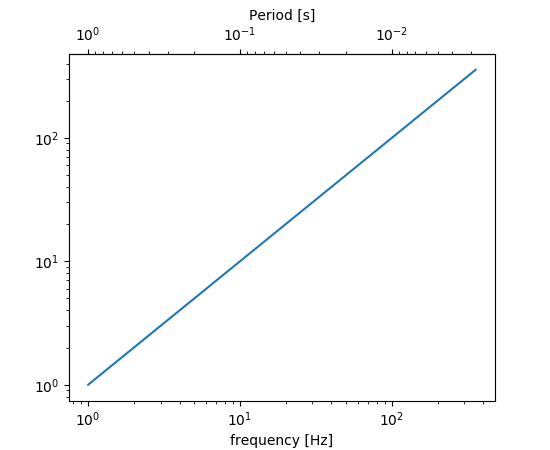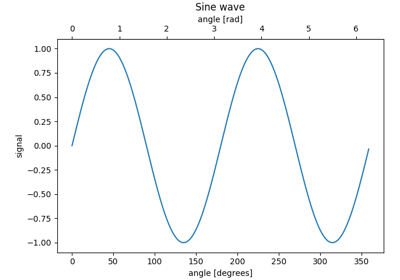matplotlib.axes.Axes.secondary_xaxis¶

Axes.secondary_xaxis(self, location, *, functions=None, **kwargs)[source]

Add a second x-axis to this axes.

For example if we want to have a second scale for the data plotted on the xaxis.

Parameters: location : string or scalar The position to put the secondary axis. Strings can be 'top' or 'bottom', for x-oriented axises or 'left' or 'right' for y-oriented axises or a scalar can be a float indicating the relative position on the axes to put the new axes (0 being the bottom (left), and 1.0 being the top (right).) functions : 2-tuple of func, or Transform with an inverse If a 2-tuple of functions, the user specifies the transform function and its inverse. i.e. functions=(lambda x: 2 / x, lambda x: 2 / x) would be an reciprocal transform with a factor of 2. The user can also directly supply a subclass of transforms.Transform so long as it has an inverse. See Secondary Axis for examples of making these conversions. ax : axes._secondary_axes.SecondaryAxis **kwargs : Axes properties. Other miscellaneous axes parameters.

Warning

This method is experimental as of 3.1, and the API may change.

Examples

The main axis shows frequency, and the secondary axis shows period.Examples using matplotlib.axes.Axes.secondary_xaxis¶Secondary Axis